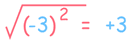# Exponents of Negative Numbers

## Squaring Removes Any Negative

"Squaring" means to multiply a number by itself.

• Squaring a positive number gets a positive result: (+5) × (+5) = +25
• Squaring a negative number also gets a positive result: (−5) × (−5) = +25

Because a negative times a negative gives a positive. So:"So what?" you say ...

... well take a look at this:Oh no! We started with minus 3 and ended with plus 3.

When we square a number, then take the square root, we may not end up with the number we started with!

In fact we end up with the absolute value of the number:

√(x2) = |x|

That also happens for all even (but not odd) Exponents.

Try here:

images/exponent-calc.js

## Even Exponents of Negative Numbers

An even exponent always gives a positive (or 0) result.

That simple fact can make our life easier:

1 (Odd):(−1)1 = −1
2 (Even):(−1)2 = (−1) × (−1) = +1
3 (Odd):(−1)3 = (−1) × (−1) × (−1) = −1
4 (Even):(−1)4 = (−1) × (−1) × (−1) × (−1) = +1

Do you see the −1, +1, −1, +1 pattern?

(−1)odd = −1

(−1)even = +1

So we can "shortcut" some calculations, like:

97 is odd, so:

(−1)97 = −1

### Example: What is (−2)6 ?

26 = 64, and 6 is even, so:

(−2)6 = +64

## Roots of Negative Numbers

### Example: What is the value of x here: x2 = −1

Does x=1?

1 × 1 = +1

Does x=−1?

(−1) × (−1) = +1

We can't get −1 for an answer!

It seems impossible!

Well, it is impossible using Real Numbers.

But we can do it using Imaginary Numbers.

In other words:

√−1 is not a Real Number ...

... it is an Imaginary Number

This is true for all even roots:

An Even Root of a Negative Number is Not Real

So just be careful when taking square roots, 4th roots, 6th roots, etc.

1742, 3998, 459, 3999, 460, 1743, 1093, 4000, 1094, 4001